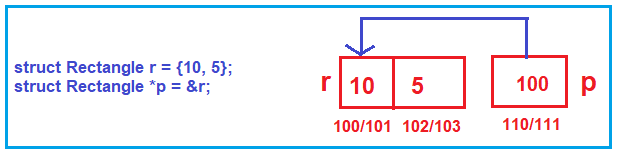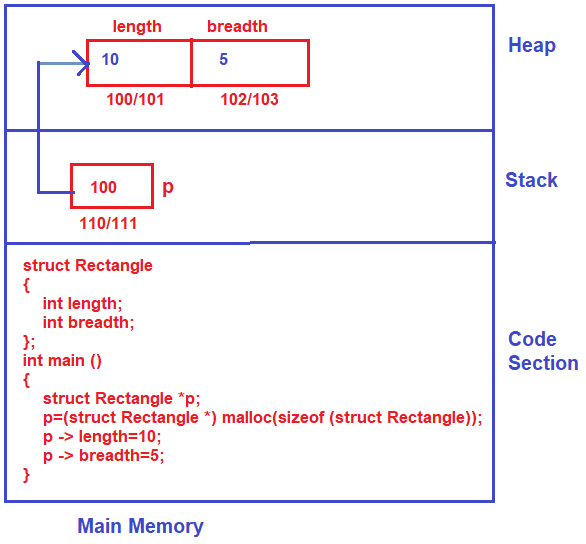# Pointer to Structure

## Pointer to Structure

In this article, I am going to discuss how to access a structure using a pointer. We have already discussed the basics of Pointers and Structures in our previous articles.

##### Let us understand this with an example

First, we will see how to access the structure using normal variables, and then we will see how to access the structure using pointer variables. Please have a look at the following code example.

```#include <stdio.h>
struct Rectangle
{
int length;
};

int main ()
{
struct Rectangle r = {10, 5};
r.length = 20;
}
```

As you can see in the above code, we have a structure Rectangle with two members i.e. length and breadth. Inside the main method, we created a data variable of type structure (struct Rectangle r = {10, 5};) and assign the length and breadth members value as 10 and 5. Then we access the structure members (length and breadth) using dot (.) operator i.e. (r.length = 20; and r.breadth = 30;).

Now, let us see how to access the structure using a pointer. So, for this first, we need to create a pointer of type structure and then we need to be initialized the pointer to the address of the structure variable as shown in the below two lines of code.As you can see, the pointer variable type is struct Rectangle, so it is going to hold the address of a structure variable. Further, if you notice the pointer variable holding the address of r.

Note Every pointer, whatever the type it has, the pointer will take 2bytes. Actually, it takes equal to the size of the integer in any compiler. If an integer takes 2bytes in the compiler pointer also takes 2bytes, if an integer takes 4bytes it will also take 4bytes. Here, we are assuming the integer takes 2 bytes,

##### How to access structure members using a pointer?

As of now, we have discussed two things. First creating a structure variable. Then creating a pointer variable of structure type and assigning it with the structure address. Now let us move and see what are the different ways to access the structure members using a pointer.

We cannot access directly using the dot operator as shown below

p.length = 20;

This is because p is a pointer, it’s not a variable. The pointer holds the address not the values directly. So, we need to go to the place where the pointer is pointing i.e. to the base address of the structure variable r, and then access the data members. To do so we need to write the pointer using * as shown below.

*p.length = 20;

Again, this is also not going to work and you will get an error. This is because the priority of the dot (.) operator is higher than the * operator. So, first, it will try to access the values and hence you will get the error. To overcome this error put *p inside a bracket and then call the structure member as shown below.

(*p).length = 20;

With the above changes in place, now it will work as expected. We have to write this much code to access the structure members. Instead of this syntax, the C programming language gives a simple syntax for accessing the members using a pointer as shown below.

p -> length = 20;

As you can see in the above, using the pointer variable and arrow (->) operator we can access the members. The complete code is given below.

```#include <stdio.h>
struct Rectangle
{
int length;
};

int main ()
{
struct Rectangle r = {10, 5};
struct Rectangle *p = &r;

p -> length = 20;

int Area = p -> length * p -> breadth;
printf("%d",Area);
}
```
##### How to get the memory in heap?

Now one more thing we will do, we will create an object dynamically in the heap using a pointer. Here, we’ll create an object or a variable of type rectangle dynamically in the Heap memory. Let us discuss this step by step.

##### Step1: Create a pointer variable

First, we need to create a pointer variable of type structure as shown below. This pointer variable is created inside the stack frame of the main memory.

struct Rectangle *p;

##### Step2: Allocating Memory in Heap

We need to create the rectangle object or variable in the heap memory. As we already discussed to create the memory in the heap we need to use the Malloc function. To the malloc function, we need to specify the size that we want in the heap memory. Here size is nothing but the size of the structure. Again, the malloc function returns a void pointer, so we need to typecast struct Rectangle pointer. The following line of code does the same.

p=(struct Rectangle *) malloc(sizeof (struct Rectangle));

The above line will allocate an object of type Rectangle in heap.

##### Step3: Accessing the Members:

Now using the arrow operator, you can access and modify the structure members as shown below.

p -> length=10;

The Complete code is given below.

```#include <stdio.h>
#include <stdlib.h>
struct Rectangle
{
int length;
};

int main ()
{
struct Rectangle *p;
p=(struct Rectangle *) malloc(sizeof (struct Rectangle));
p -> length=10;